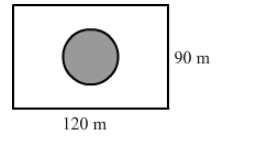# A park is in the form of a rectangle 120 m by 90 m.`
Question:

A park is in the form of a rectangle 120 m by 90 m. At the centre of the park there is a circular lawn as shown in the figure. The area of the park excluding the lawn is 2950 m2. Find the radius of the circular lawn.Solution:

Area of the rectangle $=l \times b$

$=120 \times 90$

$=10800$ sq. $\mathrm{m}$

Area of the park excluding the lawn = 2950 m2

Area of the circular lawn = Area of the park -"> Area of the park excluding the lawn

$=10800-2950$

$=7850 \mathrm{~m}^{2}$

Area of the circular lawn $=\pi r^{2}$

$\Rightarrow 7850=\frac{22}{7} \times r^{2}$

$\Rightarrow \frac{7850 \times 7}{22}=r^{2}$

$\Rightarrow r^{2}=2497.72$

$\Rightarrow r=49.97$

Or,

$r \approx 50 \mathrm{~m}$

Thus, the radius of the circular lawn is 50 m.